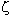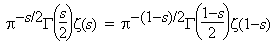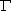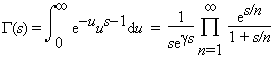# Riemann zeta function

Riemann extended the definition of Euler's zeta function(s) to all complex numbers s (except the simple pole at s=1 with residue one). Euler’s product definition of this function still holds if the real part of s is greater than one. To help understand the values for other complex numbers, Riemann derived the functional equation of the Riemann zeta function:where the gamma function(s) is the well-known extension of the factorial function ((n+1) = n! for non-negative integers n):Here the integral holds if the real part of s is greater than one, and the product holds for all complex numbers s.